# NCERT solutions for Class 8 Maths chapter 15 - Introduction to Graphs [Latest edition]

#### Chapters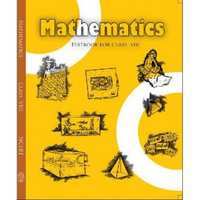## Chapter 15: Introduction to Graphs

Exercise 15.1Exercise 15.2Exercise 15.3
Exercise 15.1 [Pages 236 - 239]

### NCERT solutions for Class 8 Maths Chapter 15 Introduction to GraphsExercise 15.1 [Pages 236 - 239]

Exercise 15.1 | Q 1 | Page 236

The following graph shows the temperature of a patient in a hospital, recorded every hour.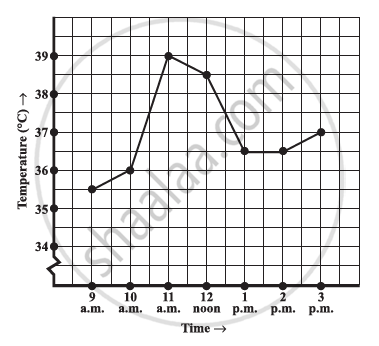1) What was the patient’s temperature at 1 p.m.?

2) When was the patient’s temperature 38.5°C?

3) The patient’s temperature was the same two times during the period given. What were these two times?

4) What was the temperature at 1.30 p.m.? How did you arrive at your answer?

5) During which periods did the patients’ temperature showed an upward trend?

Exercise 15.1 | Q 2 | Page 237

The following line graph shows the yearly sales figures for a manufacturing company.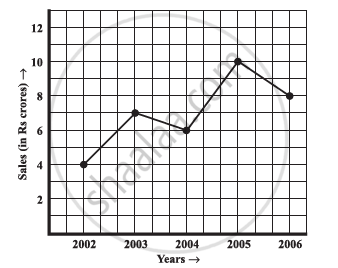1) What were the sales in (a) 2002 (b) 2006?

2) What were the sales in (a) 2003 (b) 2005?

3) Compute the difference between the sales in 2002 and 2006.

4) In which year was there the greatest difference between the sales as compared
to its previous year?

Exercise 15.1 | Q 3 | Page 237

For an experiment in Botany, two different plants, plant A and plant B were grown under similar laboratory conditions. Their heights were measured at the end of each week for 3 weeks. The results are shown by the following graph.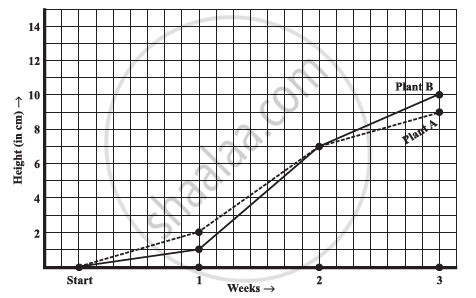1) How high was Plant A after (a) 2 weeks (b) 3weeks?

2) How high was Plant B after (a) 2 weeks (b) 3weeks?

3) How much did Plant A grow during the 3rd week?

4) How much did Plant B grow from the end of the 2nd week to the end of the 3rd week?

5) During which week did Plant A grow most?

6) During which week did Plant B grow least?

7) Were the two plants of the same height during any week shown here? Specify.

Exercise 15.1 | Q 4 | Page 238

The following graph shows the temperature forecast and the actual temperature for each day of a week.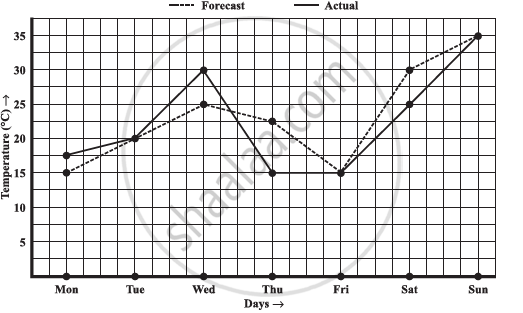1) On which days was the forecast temperature the same as the actual temperature?

2) What was the maximum forecast temperature during the week?

3) What was the minimum actual temperature during the week?

4) On which day did the actual temperature differ the most from the forecast temperature?

Exercise 15.1 | Q 5.1 | Page 238

Use the tables below to draw linear graphs

The number of days a hill side city received snow in different years.

 Year 2003 2004 2005 2006 Days 8 10 5 12
Exercise 15.1 | Q 5.2 | Page 238

Use the tables below to draw linear graphs.

Population (in thousands) of men and women in a village in different years.

 Year 2003 2004 2005 2006 2007 Number of men 12 12.5 13 13.2 13.5 Number of women 11.3 11.9 13 13.6 12.8
Exercise 15.1 | Q 6 | Page 239

A courier-person cycles from a town to a neighboring suburban area to deliver a parcel to a merchant. His distance from the town at different times is shown by the following graph.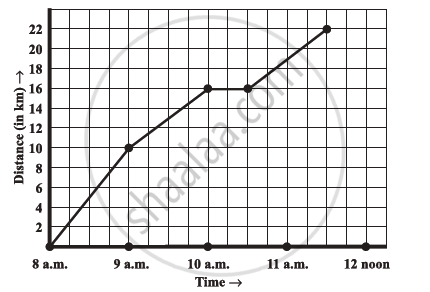1) What is the scale taken for the time axis?

2) How much time did the person take for the travel?

3) How far is the place of the merchant from the town?

4) Did the person stop on his way? Explain.

5) During which period did he ride fastest?

Exercise 15.1 | Q 7.1 | Page 239

Can there be a time temperature graph as follows? Justify you’re answer: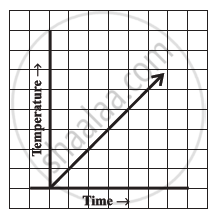Exercise 15.1 | Q 7.2 | Page 239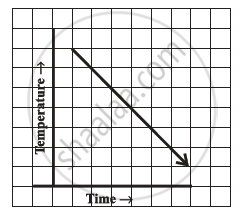Exercise 15.1 | Q 7.3 | Page 239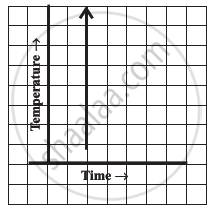Exercise 15.1 | Q 7.4 | Page 239

Can there be a time temperature graph as follows? Justify you’re answer: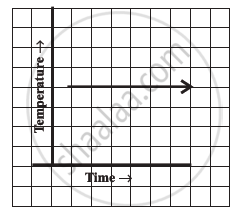Exercise 15.2 [Page 243]

### NCERT solutions for Class 8 Maths Chapter 15 Introduction to GraphsExercise 15.2 [Page 243]

Exercise 15.2 | Q 1.1 | Page 243

Plot the following points on a graph sheet. Verify if they lie on a line

A(4, 0), B(4, 2), C(4, 6), D(4, 2.5)

Exercise 15.2 | Q 1.2 | Page 243

Plot the following points on a graph sheet. Verify if they lie on a line

P(1, 1), Q(2, 2), R(3, 3), S(4, 4)

Exercise 15.2 | Q 1.3 | Page 243

Plot the following points on a graph sheet. Verify if they lie on a line

K(2, 3), L(5, 3), M(5, 5), N(2, 5)

Exercise 15.2 | Q 2 | Page 243

Draw the line passing through (2, 3) and (3, 2). Find the coordinates of the points at which this line meets the x-axis and y-axis.

Exercise 15.2 | Q 3 | Page 243

Write the coordinates of the vertices of each of these adjoining figures.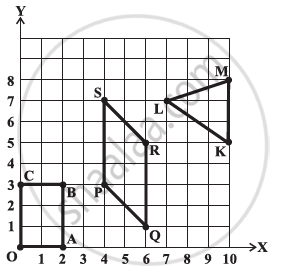Exercise 15.2 | Q 4.1 | Page 243

State whether True or False. Correct those are false.

A point whose x coordinate is zero and y-coordinate is non-zero will lie on the y-axis.

• True

• False

Exercise 15.2 | Q 4.2 | Page 243

State whether True or False. Correct those are false.

A point whose y coordinate is zero and x-coordinate is 5 will lie on y-axis.

• True

• False

Exercise 15.2 | Q 4.3 | Page 243

State whether True or False. Correct those are false.

The coordinates of the origin are (0, 0).

• True

• False

Exercise 15.3 [Pages 247 - 248]

### NCERT solutions for Class 8 Maths Chapter 15 Introduction to GraphsExercise 15.3 [Pages 247 - 248]

Exercise 15.3 | Q 1.1 | Page 247

Draw the graphs for the following tables of values, with suitable scales on the axes.

Cost of apples

 Number of apples 1 2 3 4 5 Cost (in Rs) 5 10 15 20 25
Exercise 15.3 | Q 1.2 | Page 247

Draw the graphs for the following tables of values, with suitable scales on the axes.

Distance travelled by a car

 Time (in hours) 6 a.m 7 a.m 8 a.m 9 a.m Distance (in km) 40 80 120 160

1)  How much distance did the car cover during the period 7.30 a.m. to 8 a.m.?

2) What was the time when the car had covered a distance of 100 km since its start?

Exercise 15.3 | Q 1.3 | Page 247

Draw the graphs for the following tables of values, with suitable scales on the axes.

Interest on deposits for a year:

 Deposit (in Rs) 1000 2000 3000 4000 5000 Simple interest (in Rs) 80 160 240 320 400

1) Does the graph pass through the origin?

2) Use the graph to find the interest on Rs 2500 for a year:

3) To get an interest of Rs 280 per year, how much money should be deposited?

Exercise 15.3 | Q 2.1 | Page 248

Draw a graph for the following.

 Side of square (in cm) 2 3 3.5 5 5 Perimeter (in cm) 8 12 14 20 24

Is it a linear graph?

Exercise 15.3 | Q 2.2 | Page 248

Draw a graph for the following.

 Side of square (in cm) 2 3 4 5 6 Area (in cm2) 4 9 16 25 36

Is it a linear graph?

## Chapter 15: Introduction to Graphs

Exercise 15.1Exercise 15.2Exercise 15.3## NCERT solutions for Class 8 Maths chapter 15 - Introduction to Graphs

NCERT solutions for Class 8 Maths chapter 15 (Introduction to Graphs) include all questions with solution and detail explanation. This will clear students doubts about any question and improve application skills while preparing for board exams. The detailed, step-by-step solutions will help you understand the concepts better and clear your confusions, if any. Shaalaa.com has the CBSE Class 8 Maths solutions in a manner that help students grasp basic concepts better and faster.

Further, we at Shaalaa.com provide such solutions so that students can prepare for written exams. NCERT textbook solutions can be a core help for self-study and acts as a perfect self-help guidance for students.

Concepts covered in Class 8 Maths chapter 15 Introduction to Graphs are Concept of Bar Graph, Concept of Pie Graph (Or a Circle-graph), Concept of a Line Graph, Graphical Representation of Data as Histograms, Linear Graphs, Linear Graphs, Interpretation of Bar Graphs, Drawing a Bar Graph, Some Application of Linear Graphs, Concept of Double Bar Graph, Interpretation of a Double Bar Graph, Drawing a Double Bar Graph.

Using NCERT Class 8 solutions Introduction to Graphs exercise by students are an easy way to prepare for the exams, as they involve solutions arranged chapter-wise also page wise. The questions involved in NCERT Solutions are important questions that can be asked in the final exam. Maximum students of CBSE Class 8 prefer NCERT Textbook Solutions to score more in exam.

Get the free view of chapter 15 Introduction to Graphs Class 8 extra questions for Class 8 Maths and can use Shaalaa.com to keep it handy for your exam preparation